# 同事想用递归，被我一个深度遍历打断施法

24,844

## 深度遍历

• 以一个未被访问过的顶点作为起始点，沿着顶点的边一直向下查找未被访问过的点
• 当查找不到可以访问的点，则返回上一个顶点，继续向下查找，直到所有的点都被访问过### 图解过程

``````{
value: 'A',
children: [
{
value: 'B',
children: [
{
value: 'D',
children: [
{
value: 'H',
children: []
}
]
},
{
value: 'E',
children: []
}
]
},
{
value: 'C',
children: [
{
value: 'F',
children: []
},
{
value: 'G',
children: []
}
]
}
]
}
``````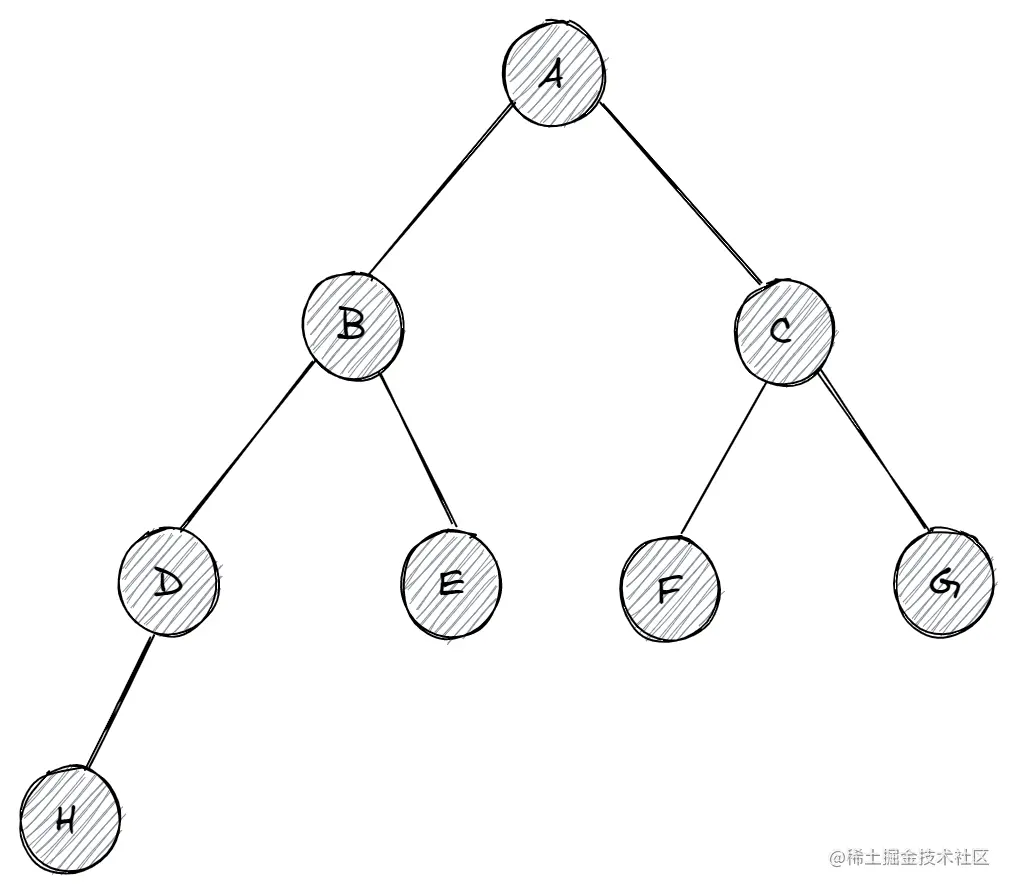#### 1. 此时从顶点A开始遍历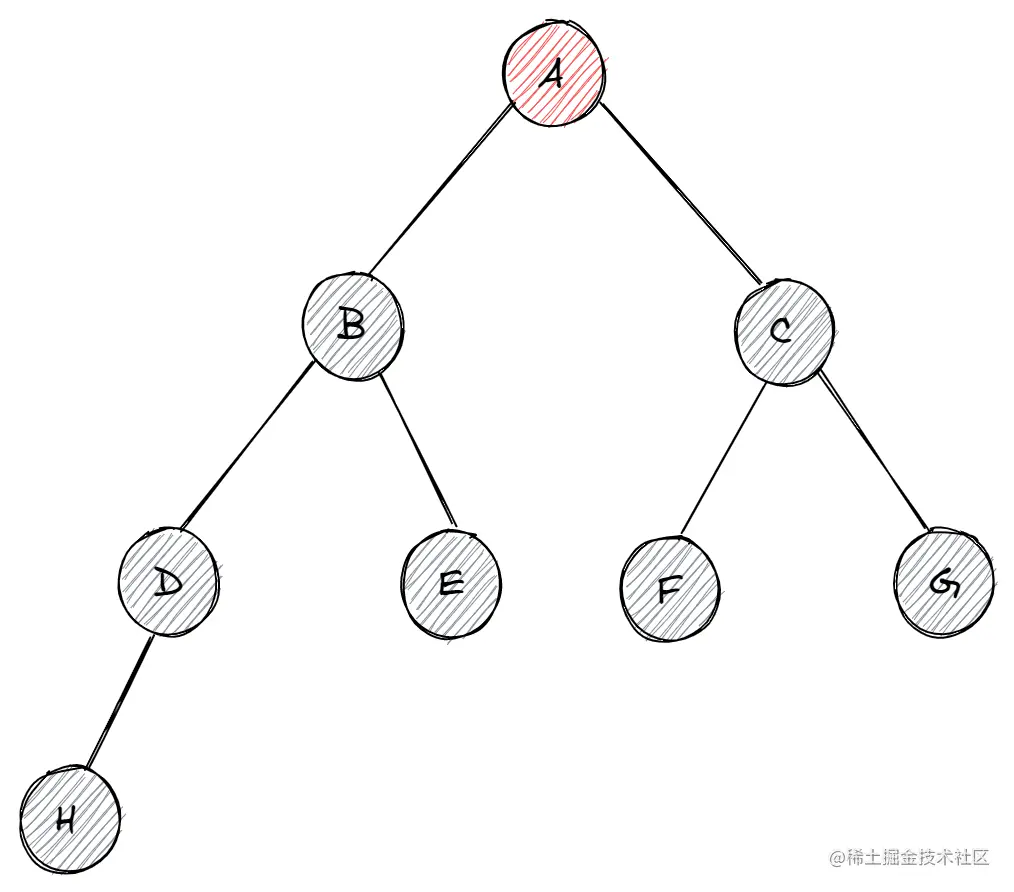A进栈，栈顶代表当前顶点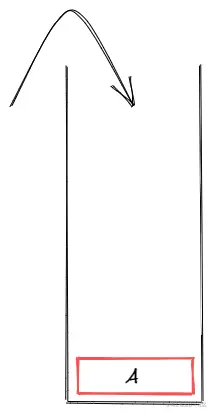#### 2. 向下查找到B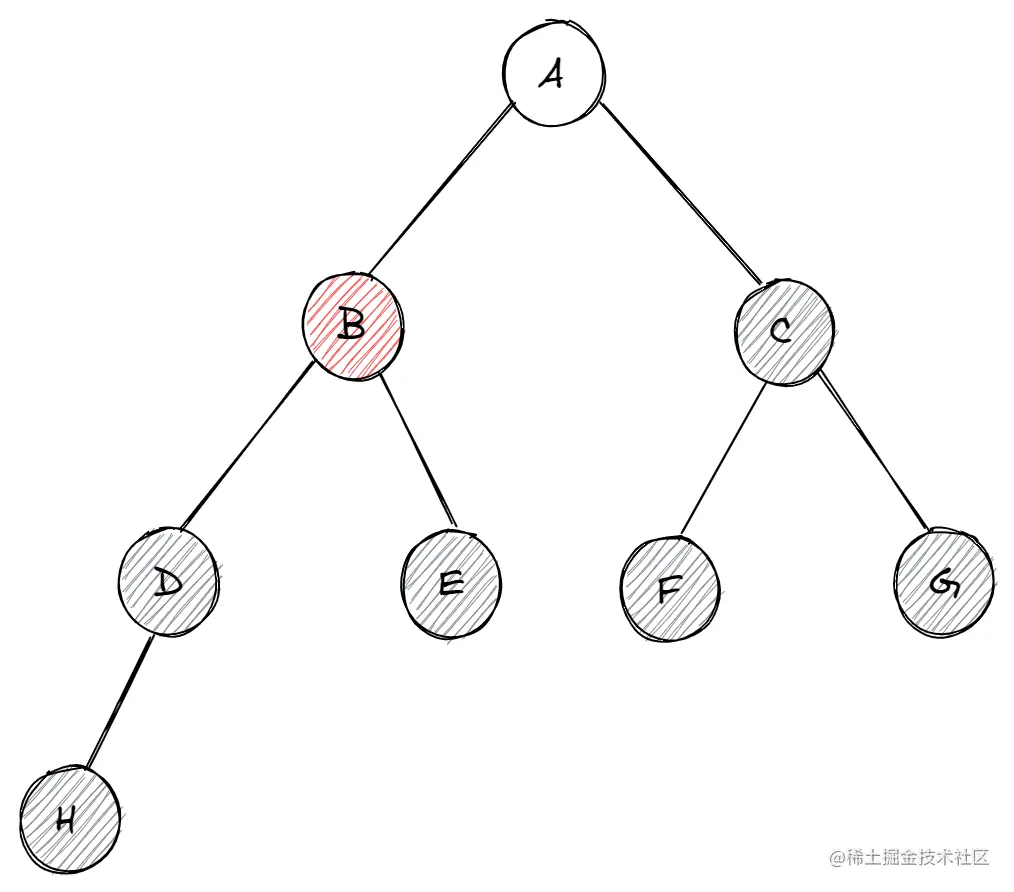A出栈，B、C进栈

A遍历后，代表已访问，栈中不需要存放，出栈。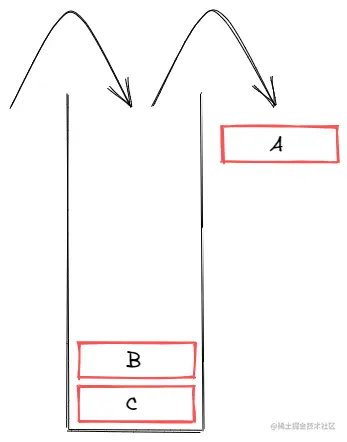#### 3. 向下查找到D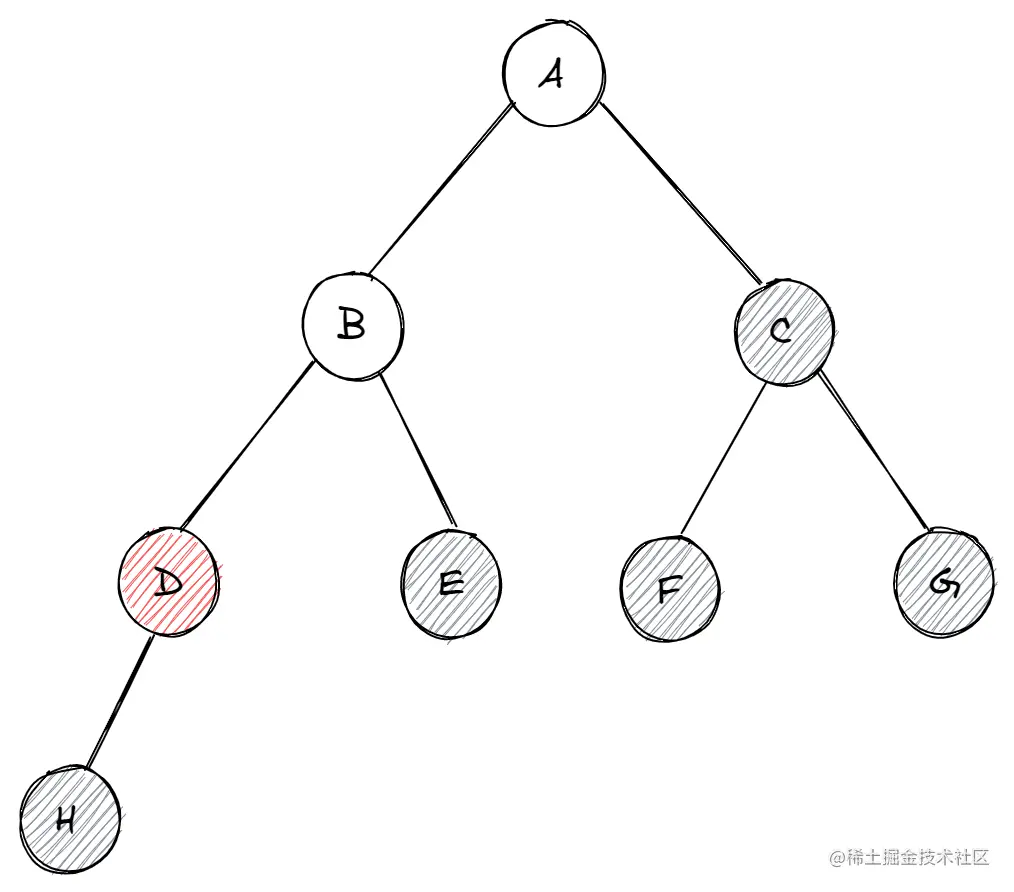B出栈，D、E进栈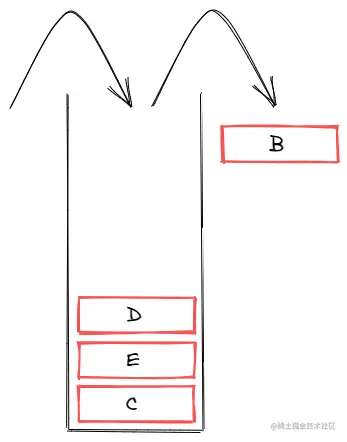#### 4. 向下查找到H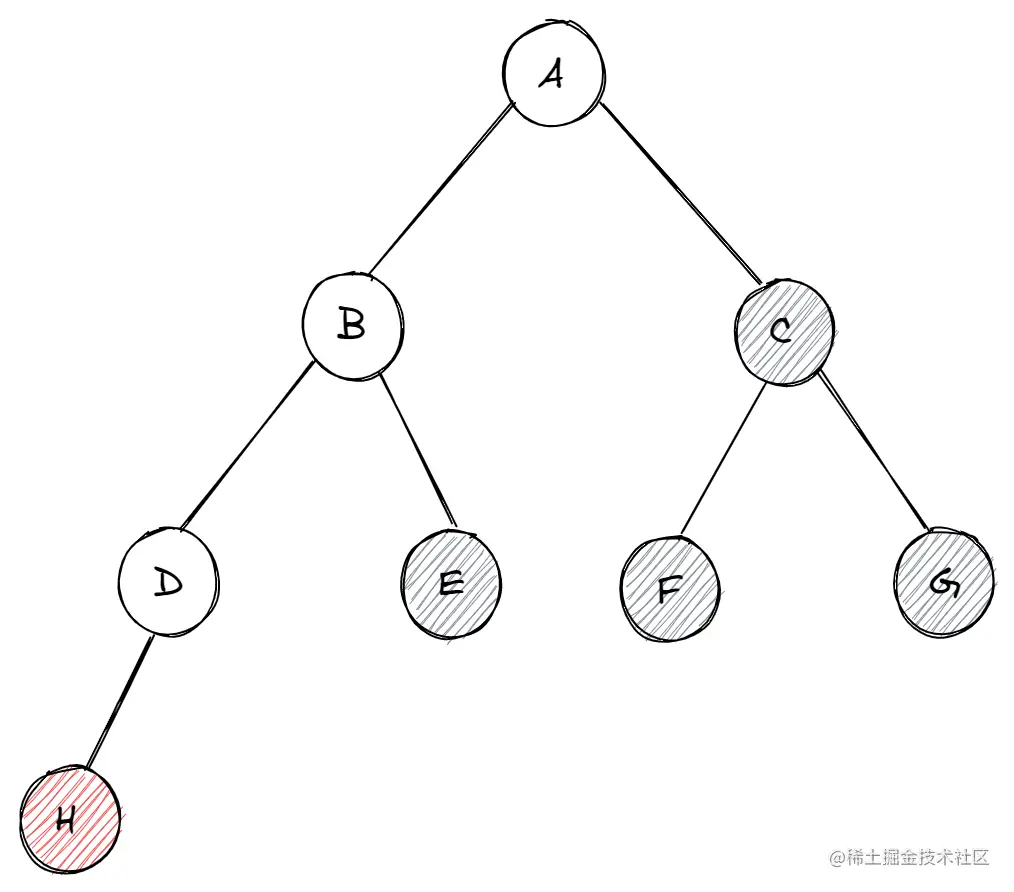D出栈，H进栈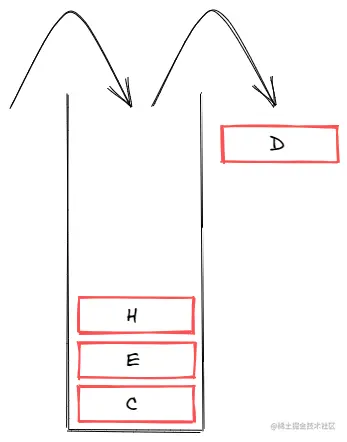#### 5. 向下无结点，返回上一个顶点E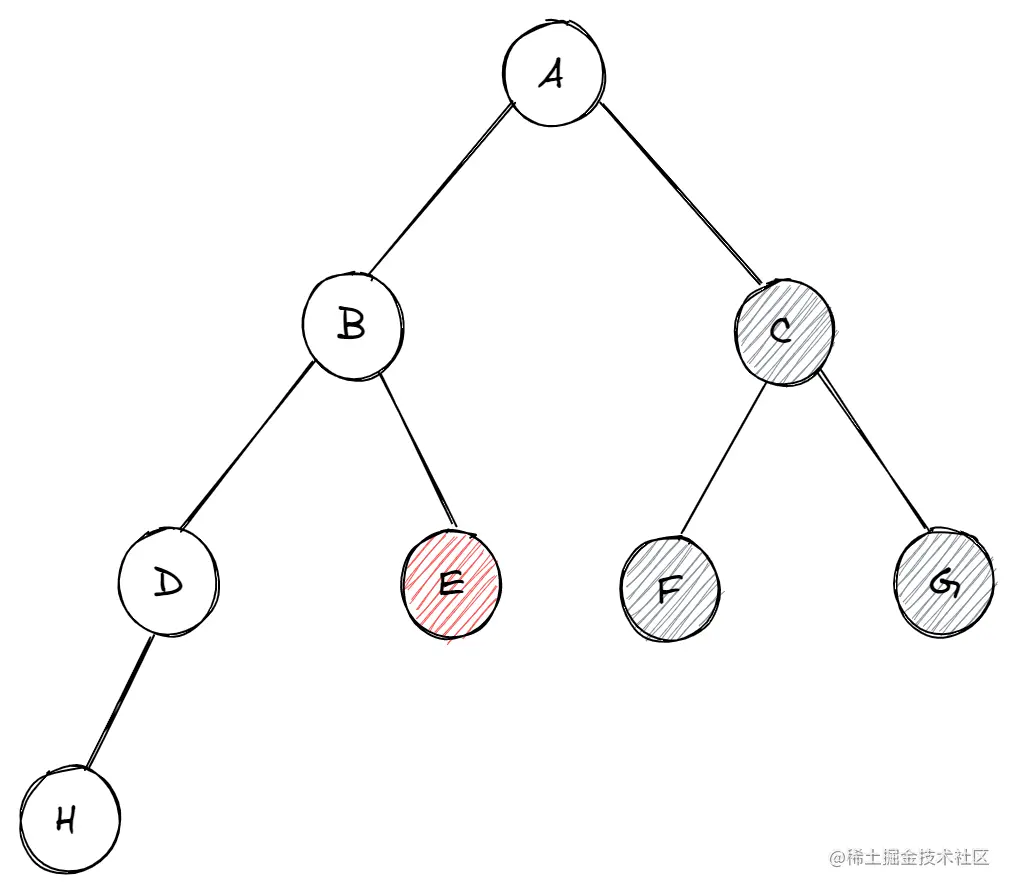H出栈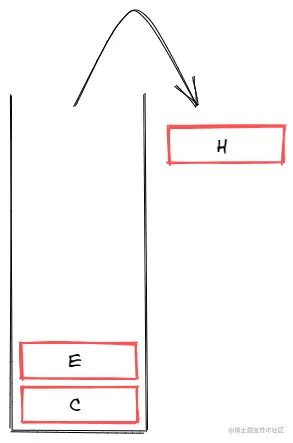#### 6. 向下无结点，返回上一个顶点C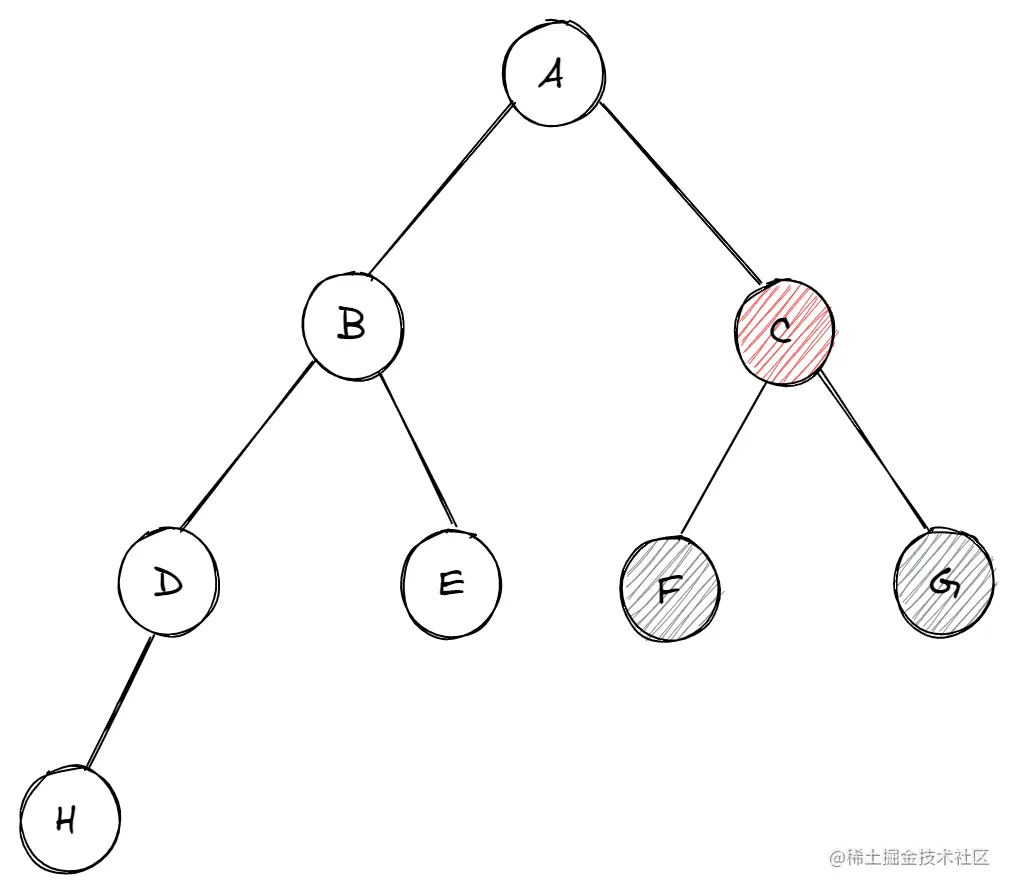E出栈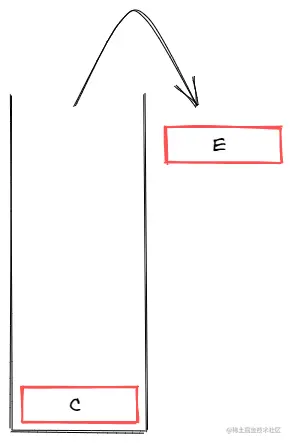#### 7. 向下查找到F结点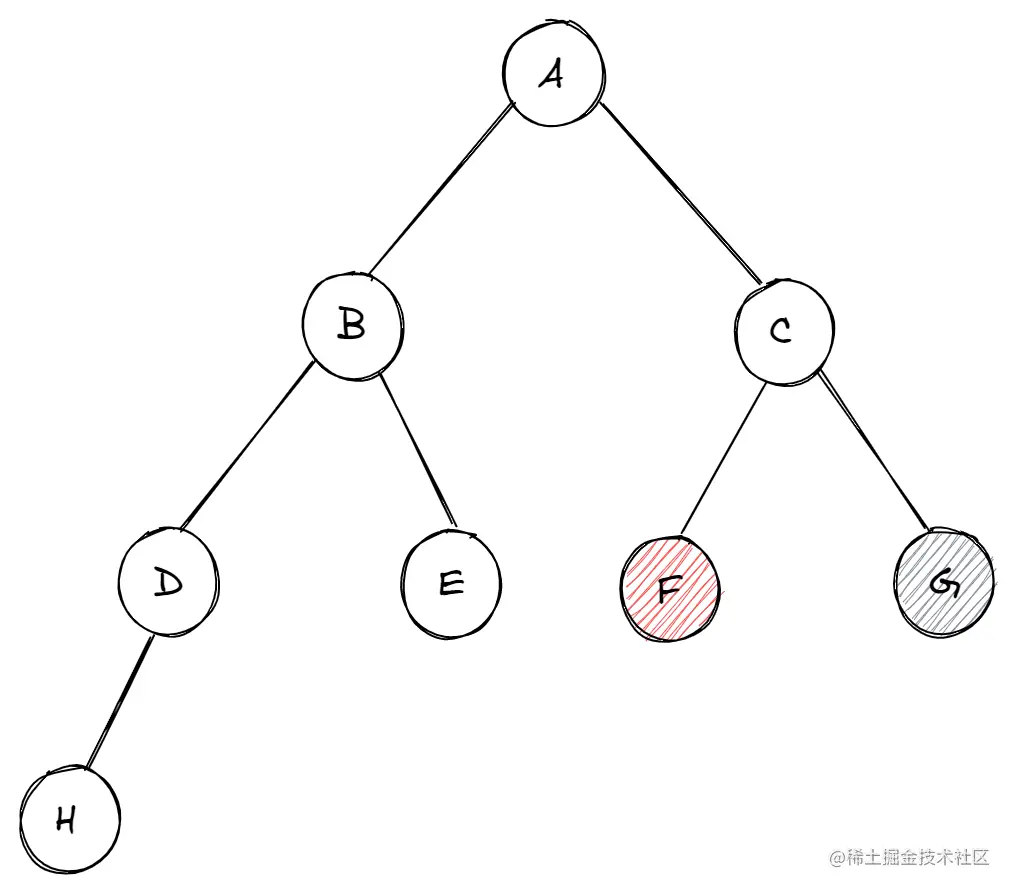C出栈，F、G进栈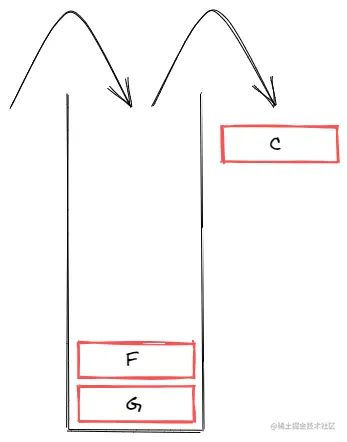#### 8. 向下无结点，返回上一个顶点G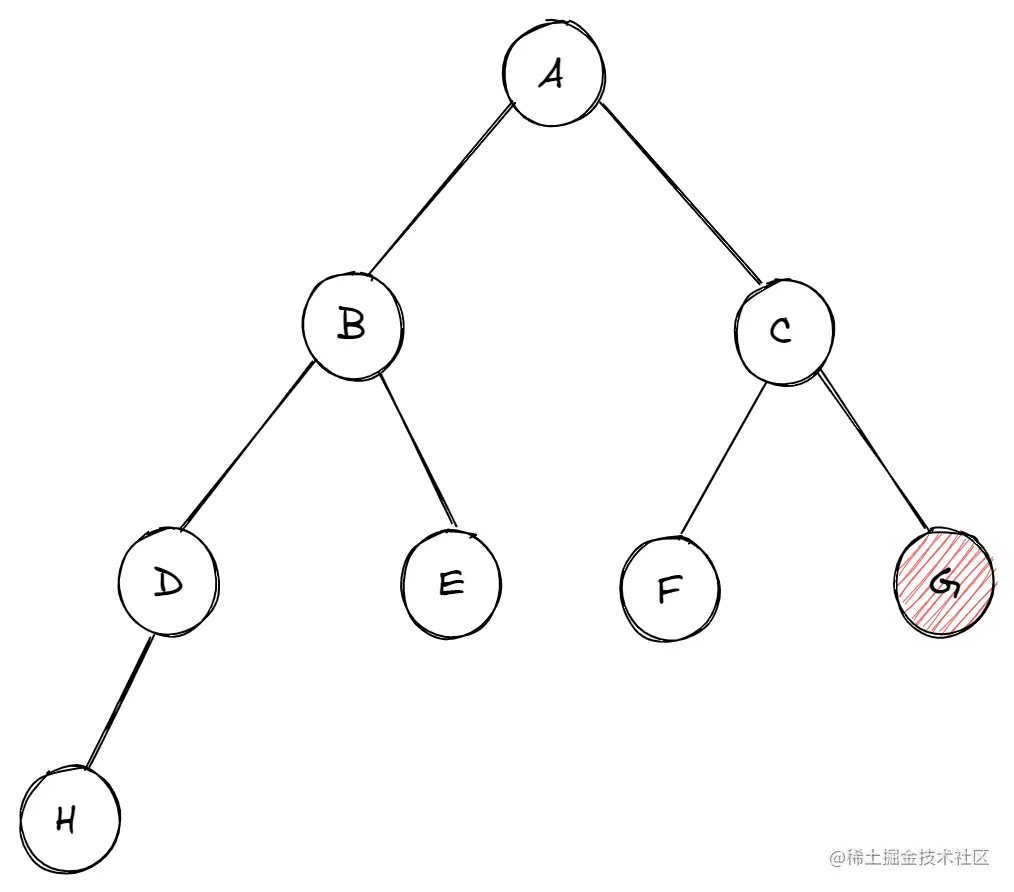F出栈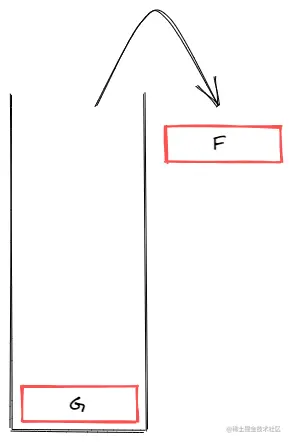#### 9. 遍历结束

G出栈，栈空，向下查找无结点，整个遍历过程结束。

### JS实现

``````/**
* 深度遍历查找
* @param {*} tree 树形数据
* @param {*} target 想要查找的目标
*/
function DFS (tree, target) {
// 模拟栈，管理结点
let stack = [tree]
while (stack.length) {
// 栈顶节点出栈
let node = stack.pop()
// 查找到目标，退出
if (node.value === target) {
return target
}
if (node.children && node.children.length) {
// 将候选顶点入栈，进行下一次循环
stack.push(...node.children.reverse())
}
}
}
``````

`reverse` 是为了遵循深度遍历的思想，沿着边向下遍历。回想上面的流程图，A遍历完后，下一个节点是B，如果这里不进行反转，直接`push`，栈内就会变成`[B, C]`，从栈顶取出的结点将会是C。

## 解决问题

• 不需要查找结点，而是全部遍历找出题目子级，遍历过程不设置目标终止条件
• 不需要强行遵循沿边遍历，即不用`reverse`
• 栈会将已访问的数据出栈，需要另建一个数组存储所需的数据

``````/**
* 查找题组下的所有题目
* @param {*} tree 树形数据
*/
function findQuestions (tree) {
// 模拟栈，管理结点
let stack = [tree]
let res = []
while (stack.length) {
// 栈顶结点出栈
let node = stack.pop()
// 题组有题目
if (node.question_list && node.question_list.length) {
// 存储需要的数据
res.push(...node.question_list)
}
// 题组下还有组
if (node.sub_group_list && node.sub_group_list.length) {
// 将候选顶点入栈，进行下一次循环
stack.push(...node.sub_group_list)
}
}
return res
}
``````